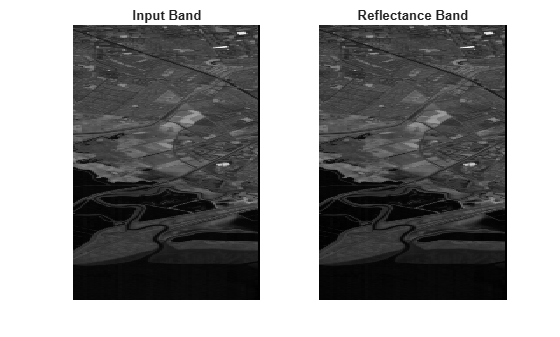# dn2reflectance

Convert digital number to reflectance

## Syntax

``newhcube = dn2reflectance(hcube)``
``newhcube = dn2reflectance(hcube,'BlockSize',blocksize)``

## Description

example

````newhcube = dn2reflectance(hcube)` converts the pixel values of the hyperspectral data cube from digital number (DN) to reflectance values. The function returns a new `hypercube` object and the pixel values of the data cube are the top of atmosphere (TOA) reflectance values. For details on TOA reflectance values, see Compute TOA Reflectance values from DNs.```
````newhcube = dn2reflectance(hcube,'BlockSize',blocksize)` specifies the block size for block processing of the hyperspectral data cube by using the name-value pair argument `'BlockSize'`.The function divides the input image into distinct blocks, processes each block, and then concatenates the processed output of each block to form the output matrix. Hyperspectral images are multi-dimensional data sets that can be too large to fit in system memory in their entirety. This can cause the system to run out of memory while running the `dn2reflectance` function. If you encounter such an issue, perform block processing by using this syntax.For example, `dn2reflectance(hcube,'BlockSize',[50 50])` divides the input image into non-overlapping blocks of size 50-by-50 and then computes the reflectance values for pixels in each block. NoteTo perform block processing by specifying the `'BlockSize'` name-value pair argument, you must have MATLAB® R2021a or a later release. NoteThis function requires the Image Processing Toolbox™ Hyperspectral Imaging Library. You can install the Image Processing Toolbox Hyperspectral Imaging Library from Add-On Explorer. For more information about installing add-ons, see Get and Manage Add-Ons. ```

## Examples

collapse all

Read hyperspectral data into the workspace.

`hcube = hypercube('EO1H0440342002212110PY_cropped.hdr');`

Determine the bad spectral band numbers using the `BadBands` parameter in the metadata.

`bandNumber = find(~hcube.Metadata.BadBands);`

Remove the bad spectral bands from the data cube.

`hcube = removeBands(hcube,'BandNumber',bandNumber);`

Convert digital numbers to top of atmosphere (TOA) reflectances. The pixel values in the output data cube are the TOA reflectances.

`newhcube = dn2reflectance(hcube);`

Read and display the 80th spectral band image in the input and the output reflectance data cubes.

```inputBand = hcube.DataCube; reflectanceBand = newhcube.DataCube; band = 80; figure subplot(1,2,1) imagesc(inputBand(:,:,band)) title('Input Band') axis off subplot(1,2,2) imagesc(reflectanceBand(:,:,band)) title('Reflectance Band') axis off colormap gray ```## Input Arguments

collapse all

Input hyperspectral data, specified as a `hypercube` object. The `DataCube` property of the `hypercube` object stores the hyperspectral data cube. The `MetaData` property of the `hypercube` object must contain reflectance gain values.

Size of the data blocks, specified as a 2-element vector of positive integers. The elements of the vector correspond to the number of rows and columns in each block, respectively. The size of the data blocks must be less than the size of the input image. Dividing the hyperspectral images into smaller blocks enables you process large data sets without running out of memory.

• If the `blocksize` value is too small, the memory usage of the function reduces at the cost of increased execution time.

• If the `blocksize` value is large or equal to the input image size, the execution time reduces at the cost of increased memory usage.

Example: `'BlockSize',[20 20]` specifies the size of each data block as 20-by-20.

## Output Arguments

collapse all

Output hyperspectral data, returned as a `hypercube` object. The pixel values of the data cube returned at the output specifies the top of atmosphere (TOA) reflectance values.

collapse all

### Compute TOA Reflectance values from DNs

Given a digital number (DN), the TOA reflectance is computed by using the reflectance gain (RGain) and reflectance offset (ROffset) of each spectral band in the data cube.

The reflectance gain and reflectance offset values of each spectral band are stored in the header file.

Alternatively, the TOA reflectance values can be estimated from digital numbers (DN) by using these two steps:

1. Compute the radiance values from the digital number (DN).

Gainλ and Biasλ are the gain and offset values for each spectral band (λ) respectively. The `Metadata` property of `hypercube` object contains the gain and offset values.

2. Compute the TOA reflectance values from the radiance values.

d is the earth-sun distance in astronomical units, ESUNλ is the mean solar irradiance for each spectral band, and θE is the sun elevation angle.